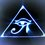# Applications of Logarithms

Richter Scale

Developed by Charles Richter in 1935 to compare the intensities of earthquakes. The amount of energy released in an earthquake is very large, so a logarithmic scale avoids the use of large numbers.

The formula used for these calculations is:

$M= \log_{10}(\frac{I}{I_0})$

Where $M$ is the magnitude on the richter scale, $I$ is the intensity of the earthquake being measured and $I_0$ is the intensity of a reference earthquake.

Let's do a quick example to clarify how this works.

The 1906 San Francisco earthquake had a magnitude of 8.3 on the Richter scale. At the same time in South America there was an eathquake with magnitude 4.1 that caused only minor damage. How many times more intense was the San Francisco earthquake than the South American one?

So because the magnitude is a base 10 log, the Richter number is actually the exponent that 10 is raised to in order to calculate the intensity of the earthquake.

So the difference in magnitudes of the earthquakes can be calculated as follows:

$M=\log_{10}(\frac{10^{8.3}}{10^{4.1}})$

$M=4.2$

You can just subtract 4.1 from 8.3 and get the same result but if your math teachers are like mine they will want you to use logarithms, and this is how it is done. The reason that subtracting the magnitudes works is because of the exponent rule for dividing exponents with the same base.

Decibel Scale

One decibel is one tenth of one bel, named in honor of Alexander Graham Bell. The bel is rarely used without the deci- prefix., deci- meaning one tenth. The decibel scale is used to calculate the difference in intensity between two sounds.

$L=10\log_{10}(\frac{I}{I_0})$

Where$L$ is the loudness of the sound measured in decibels, $I$ is the intensity of the sound being measured and $I_0$ is the intensity of the sound at the threshold of hearing which is equal to zero decibels.

$pH$ Scale

The $pH$ scale was invented in 1910 by Dr Soren Sorenson, Head of Laboratory at Carlsberg Beer Company. The "H" in $pH$ stands for hydrogen and the meaning of the "p" in $pH$, although disputed, is generally considered to mean the power of hydrogen. This scale is used to measure the acidity or alkalinity of water or water soluble substances including but definitely not limited to soil or rainwater. The $pH$ scale ranges from 1 to 14, where seven is a neutral point. Values below 7 indicate acidity with 1 being the most acidic. Values above 7 indicate alkalinity with14 being the most alkaline.

$pH=-\log_{10}[H+]$

where $pH$ is the $pH$ number from $1-14$ and $[H+]$ is the concentration of hydrogen ions.Note by Brody Acquilano
6 years, 3 months ago

This discussion board is a place to discuss our Daily Challenges and the math and science related to those challenges. Explanations are more than just a solution — they should explain the steps and thinking strategies that you used to obtain the solution. Comments should further the discussion of math and science.

When posting on Brilliant:

• Use the emojis to react to an explanation, whether you're congratulating a job well done , or just really confused .
• Ask specific questions about the challenge or the steps in somebody's explanation. Well-posed questions can add a lot to the discussion, but posting "I don't understand!" doesn't help anyone.
• Try to contribute something new to the discussion, whether it is an extension, generalization or other idea related to the challenge.

MarkdownAppears as
*italics* or _italics_ italics
**bold** or __bold__ bold
- bulleted- list
• bulleted
• list
1. numbered2. list
1. numbered
2. list
Note: you must add a full line of space before and after lists for them to show up correctly
paragraph 1paragraph 2

paragraph 1

paragraph 2

[example link](https://brilliant.org)example link
> This is a quote
This is a quote
    # I indented these lines
# 4 spaces, and now they show
# up as a code block.

print "hello world"
# I indented these lines
# 4 spaces, and now they show
# up as a code block.

print "hello world"
MathAppears as
Remember to wrap math in $$ ... $$ or $ ... $ to ensure proper formatting.
2 \times 3 $2 \times 3$
2^{34} $2^{34}$
a_{i-1} $a_{i-1}$
\frac{2}{3} $\frac{2}{3}$
\sqrt{2} $\sqrt{2}$
\sum_{i=1}^3 $\sum_{i=1}^3$
\sin \theta $\sin \theta$
\boxed{123} $\boxed{123}$

Sort by:

Out of curiosity, what does "Quit my job so I could focus on Math" refer to?

Staff - 6 years, 3 months ago

The example with earthquakes is wrong.

- 2 years, 11 months ago